# Calculating SLOPES What slope means Slope tells you

• Slides: 14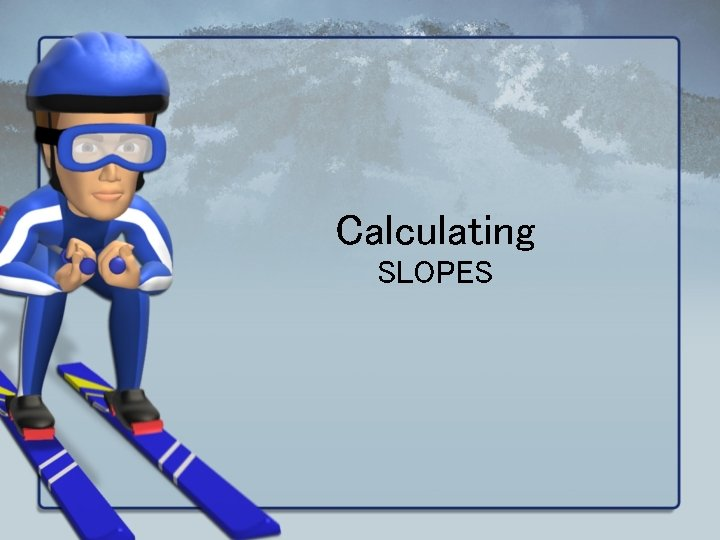Calculating SLOPES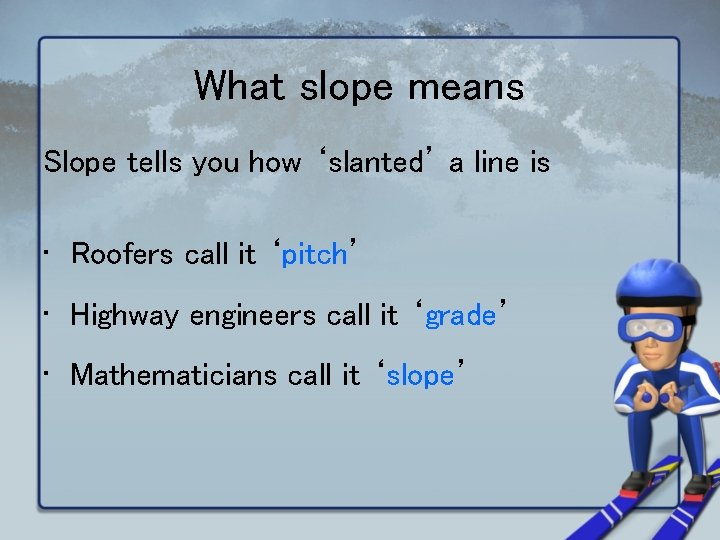What slope means Slope tells you how ‘slanted’ a line is • Roofers call it ‘pitch’ • Highway engineers call it ‘grade’ • Mathematicians call it ‘slope’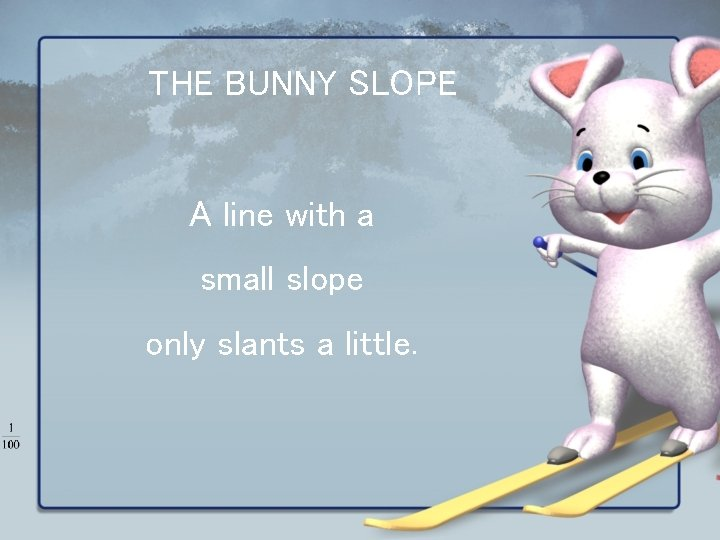THE BUNNY SLOPE A line with a small slope only slants a little.Double Black Diamond Expert Slope A line with a large slope slants a lot.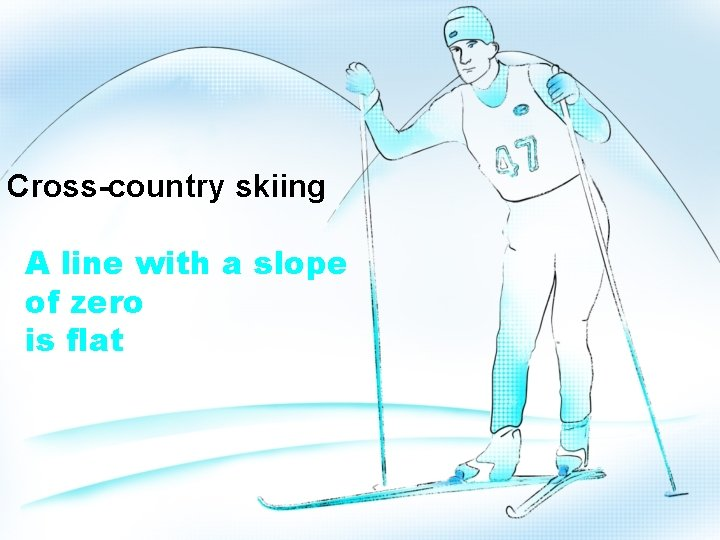Cross-country skiing A line with a slope of zero is flat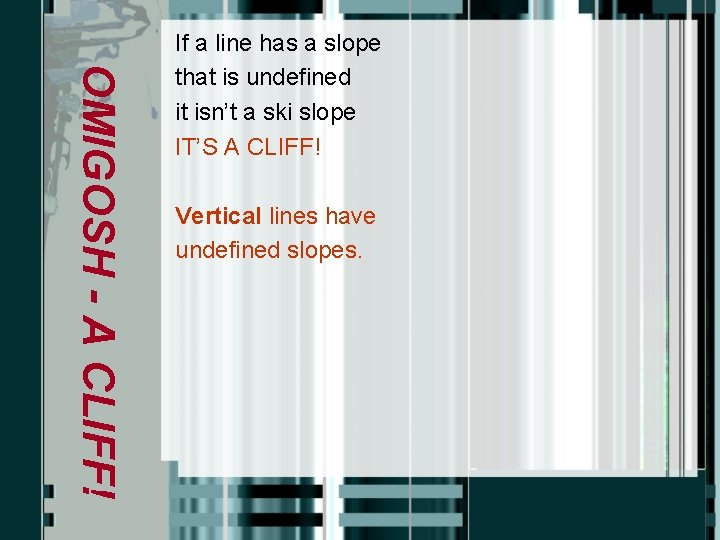OMIGOSH - A CLIFF! If a line has a slope that is undefined it isn’t a ski slope IT’S A CLIFF! Vertical lines have undefined slopes.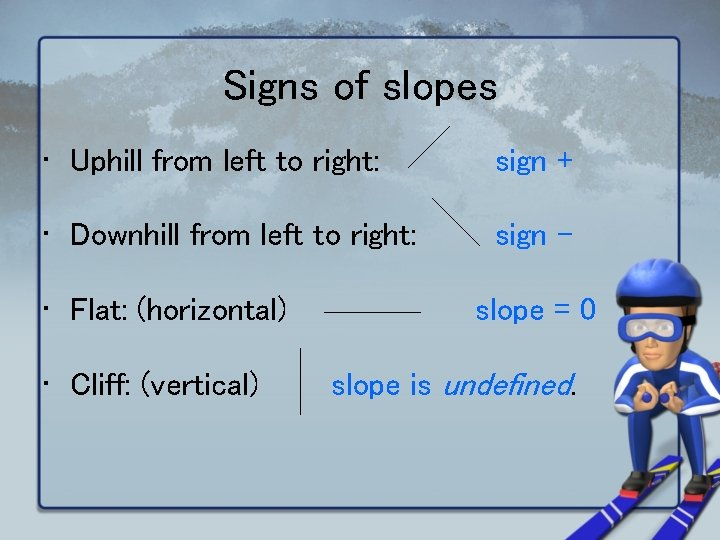Signs of slopes • Uphill from left to right: sign + • Downhill from left to right: sign – • Flat: (horizontal) • Cliff: (vertical) slope = 0 slope is undefined.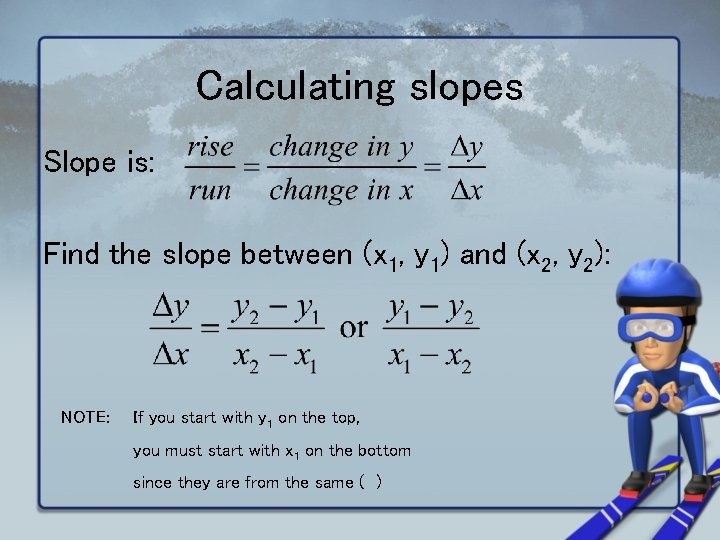Calculating slopes Slope is: Find the slope between (x 1, y 1) and (x 2, y 2): NOTE: If you start with y 1 on the top, you must start with x 1 on the bottom since they are from the same ( )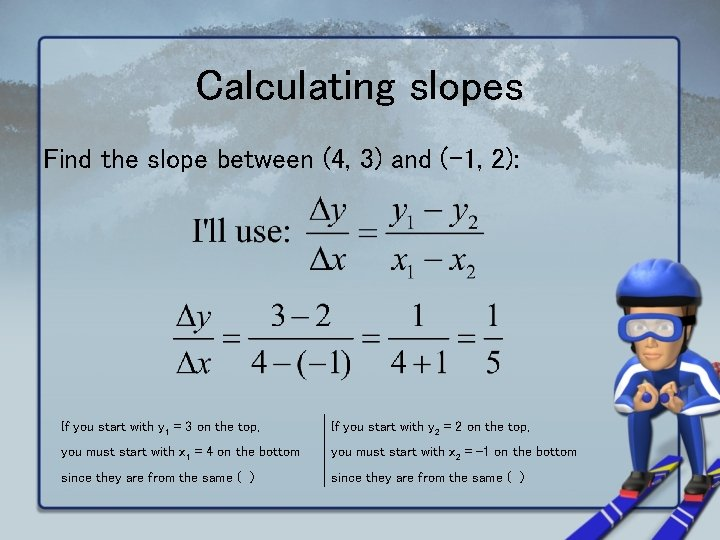Calculating slopes Find the slope between (4, 3) and (-1, 2): If you start with y 1 = 3 on the top, If you start with y 2 = 2 on the top, you must start with x 1 = 4 on the bottom you must start with x 2 = -1 on the bottom since they are from the same ( )Calculating harder slopes next. . .Calculating harder slopes next. . .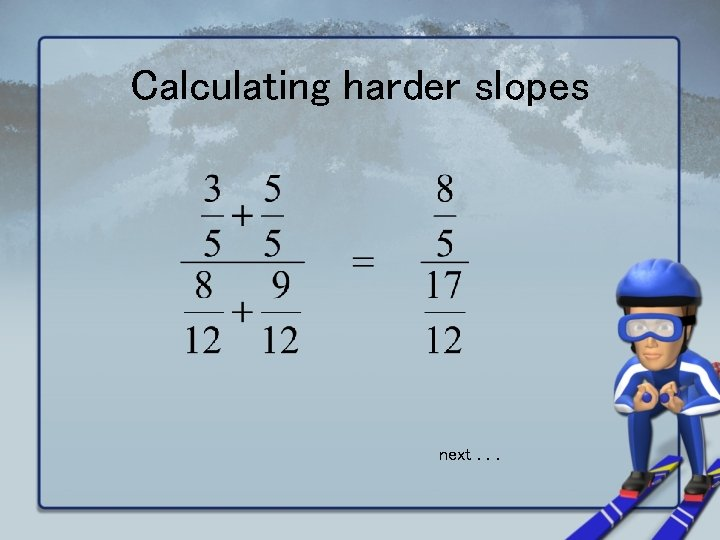Calculating harder slopes next. . .Calculating harder slopes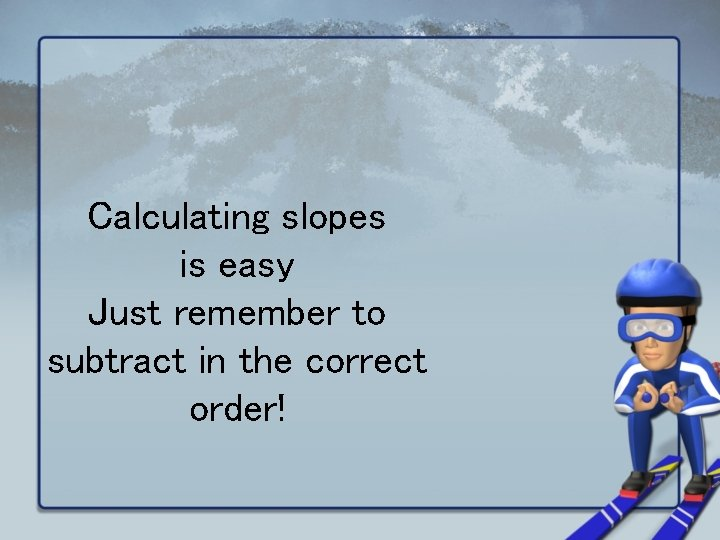Calculating slopes is easy Just remember to subtract in the correct order!